• Pytorch函数1 torch.max\ torch.min\ torch.squeeze\ torch.unsqueeze\ torch.rand \randn \randint

1.torch.max(input)

输入input:任何形状的张量

输出:返回输入张量input中所有元素的最大值 。

import torch
a = torch.randn((3,4,5))
b = torch.max(a)    # 返回所有张量中的最大值
print(a)
tensor([[[-0.5234, -2.3275,  1.8327,  0.5354,  1.1100],
[ 0.7137,  0.4375, -1.1837,  0.8168,  0.4115],
[ 1.1823, -0.3772, -0.3378,  0.0248,  0.2865],
[ 0.1679,  0.8553,  0.4570, -1.5596,  0.5309]],
[[-0.3231, -0.3478, -0.3662, -0.2204,  0.9318],
[ 0.5757, -1.7895,  0.6656, -2.1833,  0.4355],
[ 1.7979,  0.4196, -0.2651,  0.2820,  0.2115],
[-0.3352,  0.1453, -0.7170, -0.0627,  0.3263]],
[[-1.2078,  1.0757, -0.9695, -1.9247, -1.9016],
[ 1.4642,  0.2526,  1.3293,  0.7860,  0.3486],
[ 0.7677,  0.0916, -0.4585, -0.5579, -1.2199],
[-1.9069, -1.6860,  1.0152,  0.6269, -1.9427]]])
print(a.size())
torch.Size([3, 4, 5])
print(b)
tensor(1.8327)

torch.max(input, dim)

输入input:  任何形状的张量  如输入为 a = torch.randn((3,4,5))

则张量a包含三个维度(3, 4 ,5)分别对应为0 1  2三个维度

输入dim:  要压缩掉的维度    如dim=1 则输出a的形状由(3,4,5)变为(3, 5)

如dim=2  则输出a的形状由(3,4,5)变为(3, 4)

!!! dim等于几  就将几的维度压缩掉  注意是压缩掉 就是我只要这个维度的最大值  其余数据舍弃 !!!

输出out:   输出一个包含两个张量的元组,

两个张量分别是压缩指定dim后得到的最大值新张量和最大值在指定dim的索引值

c = torch.max(a,1)  # tensor a还是上边的数据,此外max(a,1)是将a的1维压缩掉.
print(a)
tensor([[[-0.5234, -2.3275,  1.8327,  0.5354,  1.1100],
[ 0.7137,  0.4375, -1.1837,  0.8168,  0.4115],
[ 1.1823, -0.3772, -0.3378,  0.0248,  0.2865],
[ 0.1679,  0.8553,  0.4570, -1.5596,  0.5309]],
[[-0.3231, -0.3478, -0.3662, -0.2204,  0.9318],
[ 0.5757, -1.7895,  0.6656, -2.1833,  0.4355],
[ 1.7979,  0.4196, -0.2651,  0.2820,  0.2115],
[-0.3352,  0.1453, -0.7170, -0.0627,  0.3263]],
[[-1.2078,  1.0757, -0.9695, -1.9247, -1.9016],
[ 1.4642,  0.2526,  1.3293,  0.7860,  0.3486],
[ 0.7677,  0.0916, -0.4585, -0.5579, -1.2199],
[-1.9069, -1.6860,  1.0152,  0.6269, -1.9427]]])
print(c)
torch.return_types.max(
values=tensor([[1.1823, 0.8553, 1.8327, 0.8168, 1.1100],
[1.7979, 0.4196, 0.6656, 0.2820, 0.9318],
[1.4642, 1.0757, 1.3293, 0.7860, 0.3486]]),
indices=tensor([[2, 3, 0, 1, 0],
[2, 2, 1, 2, 0],
[1, 0, 1, 1, 1]]))

4.torch.squeeze(input, dim=None)

输入是input,dim默认,则输出是一个将输入张量input中的所有维度中尺寸为1的维度压缩掉后的新张量,例如,如果输入为形状:(A×1×B×C×1×D)，则输出张量将为形状:(A×B×C×D),就将尺寸为1的维度都扔掉.

当dim给定时，压缩(挤压)操作只在给定的dim进行。如果输入的形状为:(A×1×B)，torch.squeeze(input, 0)将使张量保持不变，而torch.squeeze(input, 1)将把张量压缩为(A×B)。

5.torch.unsqueeze(input, dim)

即为在输入张量input的指定维度dim上增加一个尺寸为1的维度,

a = torch.randn(3,4,5)
a.size()                     # torch.Size([3, 4, 5])
a = torch.unsqueeze(a,1)     # unsqueeze 在a的1维添加一个尺寸为1的维度

a.size()                    # torch.Size([3, 1, 4, 5])
a = torch.squeeze(a)        # 将a中尺寸为1的任意维度压缩掉

a.size()            # torch.Size([3, 4, 5])

6.torch.rand(size)

返回一个和size形状的张量,张量中的数是来自[0,1)之间的随机数.

>>> torch.rand(4)
tensor([ 0.5204,  0.2503,  0.3525,  0.5673])
>>> torch.rand(2, 3)
tensor([[ 0.8237,  0.5781,  0.6879],
[ 0.3816,  0.7249,  0.0998]])

6.torch.randn(size)

回一个和size形状的张量,张量中的数是来自标准正态分布的随机数，均值为0，方差为1(也称为标准正态分布)。

>>> torch.randn(4)
tensor([-2.1436,  0.9966,  2.3426, -0.6366])

6.torch.randint(low=0,high,size)

返回一个size形状的张量,该张量中的随机整数在low(包括)和high(不包括)之间生成。

>>>torch.randint(3, 10, (2, 2))
tensor([[4, 5],
[6, 7]])

展开全文python 机器学习 pytorch
• torch.min、torch.maxtorch.argmaxtorch.min、torch.maxtorch.argmaxtorch.min、torch.maxtorch.argmax

torch.max()和torch.min()是比较tensor大小的函数

x = torch.rand(1,3)
print(x)
print(torch.min(x))

y = torch.rand(2,3)
print(y)
print(torch.min(y))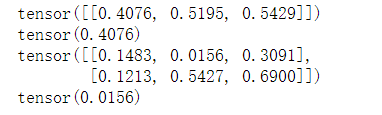指定比较维度：torch.max(input,dim)

y = torch.rand(5,3)
print("***********")
print(y)
print("***********")
print(torch.max(y,0))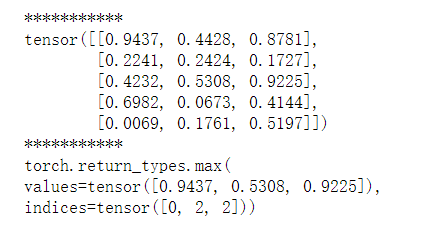y = torch.rand(5,6)
print("***********")
print(y)
print("***********")
print(torch.max(y,1))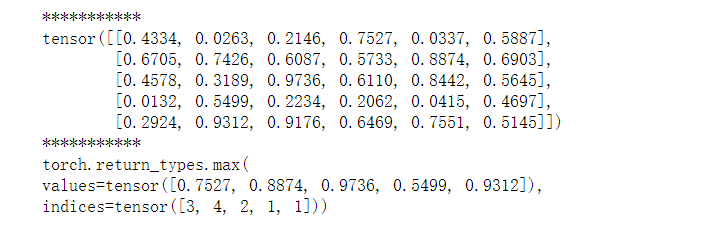两个tensor相比较：不一定是相同大小结构

若不是相同大小结构，必须满足可广播

x = torch.rand(2,3)
y = torch.rand(2,3)
print("***********")
print(x)
print("***********")
print(y)
print("*****比较结果******")
print(torch.max(x,y))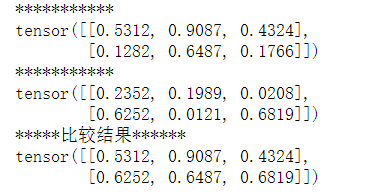展开全文• torch.max(input, dim) 返回有两个值，一个是最大值的值一个是最大值所在的下标索引 torch.argmax() 返回只有一个下标的索引 上例子： 首先创建一个tensor import torch a = torch.tensor([[1,2,3,4], [7,5...

本篇文章只讨论二维tensor，如果是多维请参考：这篇文章

torch.max(input, dim)

返回有两个值，一个是最大值的值一个是最大值所在的下标索引

torch.argmax()

返回只有一个下标的索引

上例子：

首先创建一个tensor

import torch
a = torch.tensor([[1,2,3,4], [7,5,7,4], [9,8,7,6]])

然后分别用两种方法输出：

print(torch.max(a, 1))
#print(a.max(1)) 两种格式都可以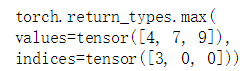print(torch.argmax(a,1))
#print(a.argmax(1))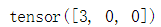展开全文python
• 在用卷积神经网络对结果进行预测时，输出的是一个Batchsize*...这时候就需要用到torch.max()函数了。 一、torch.max()、torch.min() 格式： torch.max(input, dim) 函数会返回两个tensor，第一个tensor是每...

在用卷积神经网络对结果进行预测时，输出的是一个Batchsize*n的向量。比如batchsize=64，在ImageNet是1000分类的任务。则输出的是一个64*1000的tensor。这时候，我们想要看看这每张图片的1000个预测值中哪个值最大，最大值对应的下标是多少，进而判断准确率是多少。这时候就需要用到torch.max()函数了。

一、torch.max()、torch.min()

格式：

torch.max(input, dim)

函数会返回两个tensor，第一个tensor是每行(dim=1时)的最大值；第二个tensor是每行(dim=1时)最大值的索引。【例1】

输入的imput是一个tensor，我们平时用到的就是一个batchsize*n的二维张量。一般就是想得到每行的最大值对应的结果，所以dim一般等于1，如果等于0的话就是取每一列的最大值了。【例1】【例2】。输出一般不想看最大值是多少，而是想看下标是多少，所以结果的第一个tensor一般不要。【例2】

如果不设置维度的话，会求一个全局的最大值。【例3】

例1：

import torch

outputs = torch.randn(3, 5)           #模拟batchsize=3，5分类任务的输出。
print(outputs)

print(torch.max(outputs, 1))

结果：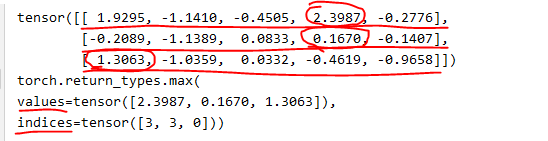例2：

import torch

outputs = torch.randn(3, 5)           #模拟batchsize=3，5分类任务的输出。
print(outputs)

_, predicted = torch.max(outputs, 1)   #取出每一行最大值对应的索引
print(predicted)

结果：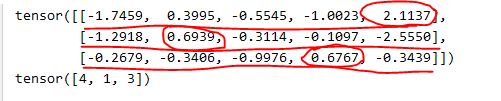例3：

import torch

outputs = torch.randn(3, 5)           #模拟batchsize=3，5分类任务的输出。
print(outputs)

res = torch.max(outputs)
print(res)

结果：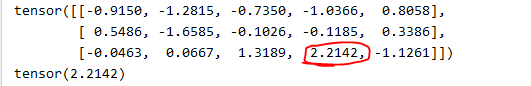二、torch.topk()

torch.topk()返回的就是前几个最大值及对应的下标了。

格式：

torch.max(input, k, dim, largest=True)

• input：一个tensor数据
• k：指明是得到前k个数据以及其index
• dim： 指定在哪个维度上排序， 默认是最后一个维度
• largest：如果为True，按照大到小排序； 如果为False，按照小到大排序

例：

import torch

outputs = torch.randn(3, 5)           #模拟batchsize=3，5分类任务的输出。
print(outputs)

_, predicted = torch.topk(outputs, k=2, dim=1)   #取出每一行最大的两个值对应的索引
print(predicted)

结果：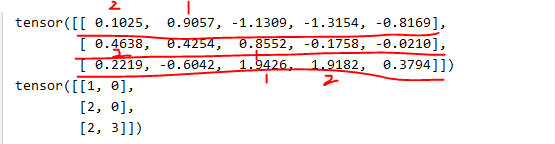展开全文•  pytorch中torch.max()和torch.min()分别表示求张量的最大值，最小值以及它们对应的索引。 torch.max(input,dim) 输入： input：表示输入的张量 dim：表示的是索引的维度，0和1分别表示列和行 输出： 返回两个...pytorch 深度学习 python
• torch.max() 和 torch.min()

千次阅读 2020-01-10 16:36:52
torch.max()和torch.min()是比较tensor大小的函数。两者用法相同，所以就总结了一个。 （1）不指定比较维度：torch.max(input) x = torch.rand(1,3) print(x) print(torch.min(x)) y = to...torch.max torch.min
• PyTorch - torch.maxtorch.min flyfish torch.max 找出输入张量中所有元素的最大值 参数 input（张量）–输入张量。 dim（int）–要减少的维。 keepdim（布尔 bool）–输出张量是否保持dim。 默认值：False。 out...
• 1、torch.max() torch.max(input,dim,keepdim=False) 返回命名元组（最大值，最大值索引），最大值是给定维度中的最大值，索引为在对应维度中的索引 当有多个最大值时，返回第一个最大值的索引 当没有指定维度 ...python
• torch.max和torch.softmax

千次阅读 2020-03-06 12:50:55
if __name__ == '__main__': import torch import torch.nn.functional as F input = torch.randn(2, 2) print(input) b = torch.softmax(input, dim=0) # 按列SoftMax,列和为1 print(b) ...
• 详解 torch.max 函数

千次阅读 2020-10-03 13:20:10
torch.max() 返回输入张量所有元素的最大值。 参数: input (Tensor) – 输入张量 例子： >>> a = torch.randn(1, 3) >>> a 0.4729 -0.2266 -0.2085 [torch.FloatTensor of size 1x3] >&...Pytorch
• Pytorch学习-torch.max和min深度解析max的使用dim参数理解二维张量使用max()三维张量使用max()小例子 max的使用 参考链接： 参考链接： 对于tensorA和tensorB： 1）torch.max(tensorA) 返回tensor中的最大值 2）...python pytorch 深度学习
• torch.max()与torch.tensor()的用法

千次阅读 2020-03-14 23:02:32
torch.max() torch.max()简单来说是返回一个tensor中的最大值。 例如： si=torch.randn(4,5) print(si) tensor([[ 1.1659, -1.5195, 0.0455, 1.7610, -0.2064], [-0.3443, 2.0483, 0.6303, 0.9475, 0.4364], ...
• argmax(f) 返回函数f的值取最大值时自变量的值 np.argmax(a) 取回对应的索引index(从下标0开始) 2.多个维度 1.np.argmax(） a = np.array([[1, 5, 5, 2], [9, 6, 2, 8], [3, 7, 9, 1]]) np.argmax(a,axis=0) ...
• pytorch: torch.max() 使用与理解

千次阅读 2021-12-13 10:40:15
predict = torch.max(outputs.data, 1) 其中 output 为模型的输出，该函数主要用来求 tensor 的最大值。 每次看到都不太理解 torch.max() 的使用，为了下次看到或者写道时不会忘记，特意详细了解其用法。 torch....pytorch 深度学习 python
• 下面讲解一下torch.max()函数的输入及输出值都是什么。 1. torch.max(input, dim) 函数 output = torch.max(input, dim) 输入 input是softmax函数输出的一个tensor dim是max函数索引的维度0/1，0是每列的最大...
• Pytorch中，经常会使用torch.max(a,dim)对tensor进行处理，特别是针对多维的tensor，就感觉对dim的选取似懂非懂。 一、针对1维的数据 这个比较好理解，就是针对1维的数据取最大值，返回一个tensor类型的数值，和该...python
• _,predicted = torch.max(outputs.data,dim)：返回最大值所在索引 predicted = torch.max(outputs.data,dim)：返回最大值 import torch tensor = torch.tensor([[1.2,-3,-6.,-9.0,-4.56,5.678]]) _,predicted = ...pytorch 深度学习
• 形式： torch.max(input, dim) 函数的输入： input： input是分类函数输出的tensor dim： dim是max函数索引的维度0/1，0是每列的最大值，1是每行的最大值 函数的输出： 函数会返回两个tensor，第一个tensor是每...pytorch 人工智能 python
• ＊的作用可以参考... torch.max可以参考https://blog.csdn.net/Z_lbj/article/details/79766690 a.size() # Out: torch.Size([6, 4, 3]) torch.max(a, 0).size() # Out[135...
• torch.max()用法

千次阅读 2020-08-18 20:15:46
版权声明：本文为CSDN博主「摇摆的果冻」的原创文章，遵循...torch.max(input) → Tensor 返回输入tensor中所有元素的最大值 a = torch.randn(1, 3) >>0.4729 -0.2266 -0.2085 torch.max(a) >>0.4729
• Pytorch中tensor维度和torch.max()函数中dim参数的理解 维度 参考了 https://blog.csdn.net/qq_41375609/article/details/106078474 ， 对于torch中定义的张量，感觉上跟矩阵类似，不过常见的矩阵是二维的。当定义...python pytorch 深度学习
• _, preds = torch.max(outputs.data, 1)

千次阅读 2021-11-22 09:08:41
今天在看《PyTorch深度学习》这本书的时候，看到了一段代码，怎么都看不懂，然后CSDN上搜索了一下，发现了大佬的以篇博客《PyTorch系列 | _, predicted = torch.max(outputs.data, 1)的理解》，这里记录一下。...pytorch
• torch.max中keepdim的作用

千次阅读 2021-01-13 17:35:37
torch.max的用法 (max, max_indices) = torch.max(input, dim, keepdim=False) 输入： 1、input 是输入的tensor。 2、dim 是索引的维度，dim=0寻找每一列的最大值，dim=1寻找每一行的最大值。 3、keepdim 表示是否...pytorch
• torch.topk, torch.max(), torch.argmax()3. unsqueeze() 1. decoder_outputs[:,t,:] = decoder_output_t decoder_outputs 形状 [batch_size, seq_len, vocab_size] decoder_output_t 形状[batch_size, vocab_s深度学习 pytorch
• torch.max()使用讲解

万次阅读 多人点赞 2020-01-07 14:34:31
torch.max(input, dim) 函数2.准确率的计算 在分类问题中，通常需要使用max()函数对softmax函数的输出值进行操作，求出预测值索引。下面讲解一下torch.max()函数的输入及输出值都是什么。 1. torch.max(input, dim)...深度学习
• PyTorch 常用方法总结2：常用Tensor运算（均值、方差、极值、线性插值……
• torch.max PyTorch中 tensor.detach() 和 tensor.data 的区别pytorch 深度学习 人工智能...